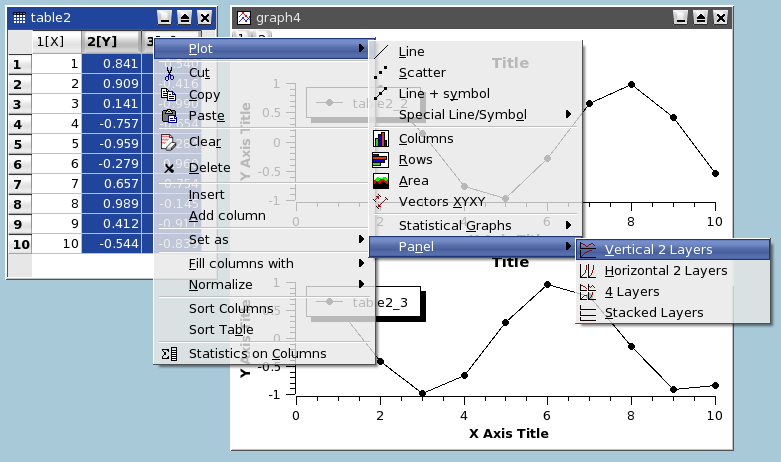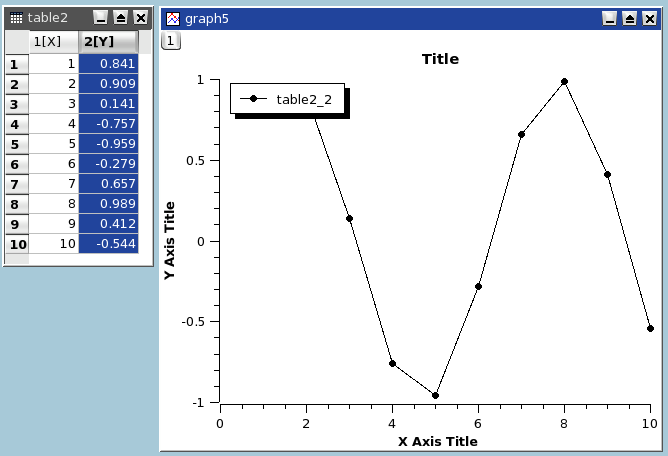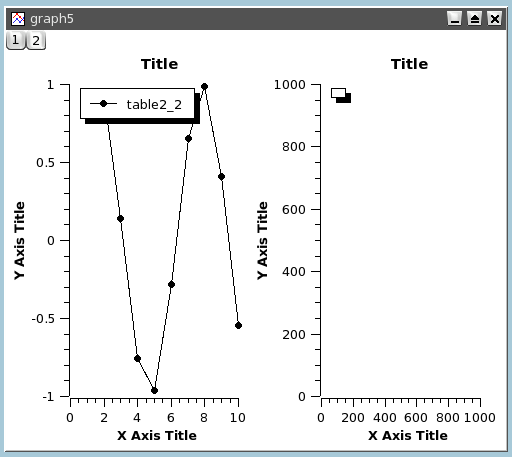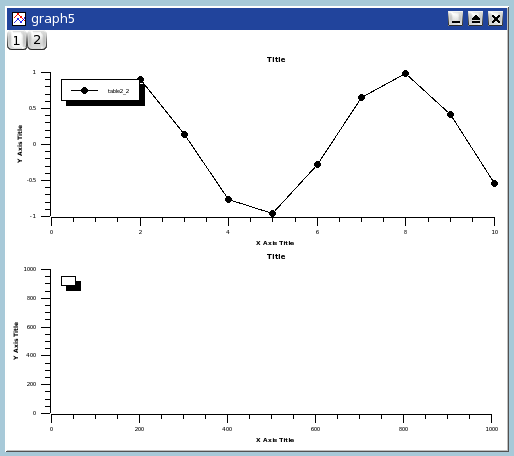# Multilayer Plots

Graph windows can contain multiple layers, each with different characteristics. Each layer has a corresponding, numbered button. A button appears pressed when its layer is the currently active layer. Only one layer is active at a time, and the plot tools (zoom, cursors, drawing tools, delete and move points) only operate on this layer. A layer is made active by clicking on it or on its corresponding button.

To arrange layers use the Arrange Layers... dialog. You can add or remove layers with the Add Layer and Remove Layer or copy/paste layers from one multilayer window to another. All these functions can be reached via the Graph menu, by using the Plot toolbar or via the context menu (right click in the multilayer window anywhere outside a layer area).

You can resize and move a layer using the Layer geometry dialog (Select the Geometry tab in Plot... from the Format Menu). You can also arrange and resize layers by hand using the mouse. First, select the layer to be modified or moved and then select the layer's plot area by left clicking on a border line (an axis line) of the plot area. QtiPlot will draw a box outlining the plot area with drag handles at the corners and midpoints of the sides. The cursor will assume a shape based upon where it is located on the layer: a hand shape when inside the plot area, or a double ended arrow when over one of the drag handles. The cursor's function, which is invoked by pressing the left mouse button, is indicated by this shape.

Pressing and holding the left mouse button when the cursor assumes the hand shape allows dragging the entire layer with the mouse. Releasing the mouse button will drop the layer at the new position. Pressing and holding the left mouse button when the cursor assumes one of the double ended arrow shapes allows dragging the corresponding border of the plot area, scaling the layer as needed. Releasing the mouse button will drop the border and apply the new scale value. Grabbing a midpoint handle moves only the corresponding border, while grabbing a corner handle allows simultaneous dragging of both corresponding borders. Dragging the corner handles does not preserve the aspect ratio of the layer.

You can also conveniently resize a layer using the mouse wheel in combination with either the Ctrl, Alt, or Shift keys. In order to use the mouse wheel, the desired layer must be selected, either by clicking in the layer or by using one of the layer selection buttons. It is not necessary to have the plot area selected, although the wheel functions will work in either case. The wheel functions work as follows: Pressing and holding the Ctrl key while rotating the wheel resizes the height, pressing and holding the Alt key while rotating the wheel resizes the width, pressing and holding the Shift key while rotating the wheel resizes both the height and width. Note that in this last case (Shift+Wheel), the aspect ratio of the layer is preserved.

## Building a multilayer plot panel

This is the simplest way to obtain a multilayer plot. It can be used if you want to build a panel of plots with a simple arrangement: 2 plot in a row or in a column, or 4 plots in 2 rows and 2 columns.

You can select two columns with Y-values in a table, and then use one of the Panel commands in the Plot menu. QtiPlot will create a panel of plots in which the size of the different elements of each plot are synchronized.You can then customize the plots. If you want to change the arrangement of the panel, use the Arrange Layers... from the Graph menu. In this case, keep in mind that each plot is in its own layer whose surface area is one half or one quarter of the window's surface area. So, if you want to share an element between two plots (for example a text label), you need to add it in a new layer (see the Add Text for more details).

## Building a multilayer plot step by step

If you need to build a more complex multilayer plot, you can define it step by step.

The first step is to build your first plot (for example from two columns of a table). Start by creating a standard graph window:Then, select the plot window and use the Add Layer from the Graph menu. This will activate the Add Layer dialog. If you choose "Guess" you will obtain a panel with two columns, if you choose "corner" you will obtain two superposed layers, you can then modify these two layers.If you want to build a panel with two rows, you can use the Arrange Layers... to automatically rearrange the layers.Then select the second (empty) plot and use the Add/Remove Curves... command to select the Y-values from one of the tables of the project.After this, you can customize your plot. At the end, the modifications done on the axis or on the axis labels may have modified the geometry of the two plots. You can again rearrange the two plots by using the Arrange Layers... a second time.